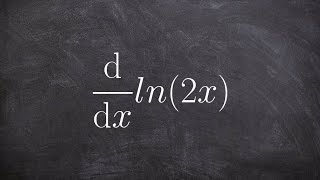# Related Videos

## Find the integral $\int x\cos\left(2x^2+3\right)dx$

Go!
Go!
1
2
3
4
5
6
7
8
9
0
a
b
c
d
f
g
m
n
u
v
w
x
y
z
.
(◻)
+
-
×
◻/◻
/
÷
2

e
π
ln
log
log
lim
d/dx
Dx
|◻|
θ
=
>
<
>=
<=
sin
cos
tan
cot
sec
csc

asin
acos
atan
acot
asec
acsc

sinh
cosh
tanh
coth
sech
csch

asinh
acosh
atanh
acoth
asech
acsch

### Videos### Using a line integral to find the work done by a vector field example | Khan Academy### Calculus - Find the derivative of natural logarithm using product property, d(ln(2x))/dx### Definite integral of rational function | AP Calculus AB | Khan Academy### _-substitution: definite integral of exponential function | AP Calculus AB | Khan Academy### Implicit differentiation | Advanced derivatives | AP Calculus AB | Khan AcademySnapXam A2

### beta Got another answer? Verify it!

Go!
1
2
3
4
5
6
7
8
9
0
a
b
c
d
f
g
m
n
u
v
w
x
y
z
.
(◻)
+
-
×
◻/◻
/
÷
2

e
π
ln
log
log
lim
d/dx
Dx
|◻|
θ
=
>
<
>=
<=
sin
cos
tan
cot
sec
csc

asin
acos
atan
acot
asec
acsc

sinh
cosh
tanh
coth
sech
csch

asinh
acosh
atanh
acoth
asech
acsch

$\int\left(x\cdot\cos\left(2x^2+3\right)\right)dx$

Calculus

~ 0.04 s